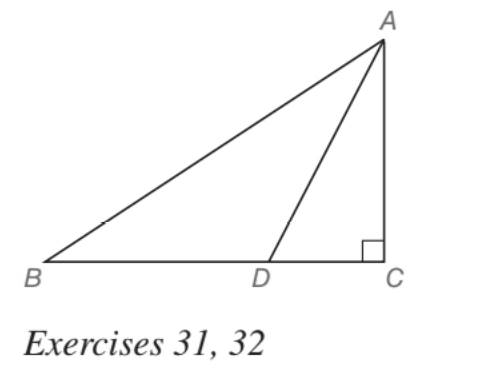Chapter 5.5, Problem 32EElementary Geometry For College St...

7th Edition
Alexander + 2 others
ISBN: 9781337614085

Solutions

Chapter
SectionElementary Geometry For College St...

7th Edition
Alexander + 2 others
ISBN: 9781337614085
Textbook Problem

In Exercises 27 to 33, give both exact solutions and approximate solutions to two decimal places. Given: Right ∆ A B C with m ∠ C = 90 ° and m ∠ B A C = 60 ° ; points D on B C ¯ ; A D ⃑ bisects ∠ B A C and A C ¯ = 2 3 Find: B DTo determine

To find:

BD in a right ABC such that mC=90° and mBAC=60°; point D on BC¯;AD

Bisects BAC and AC=23.

Explanation

Approach:

For a right triangle, for which the measure of the interior angles 30°, 60°, and 90°; if ‘a’ is the length of measure of the shorter leg; opposite to the angle 30°, then the length of the other two sides is given by

Length of the longer leg (opposite to 60°) =a3

Length of the hypotenuse (opposite to 90°)=2a.

In general

Length of the longer leg =3× (Length of the shorter leg)

Length of the hypotenuse =2× (Length of the shorter leg)

Calculation:

Given,

A right ABC,

mC=90° and mBAC=60°; point D on BC¯;AD

Bisects BAC and AC=23.

Since, AD bisects A of the ABC, we have

=12(60°)

First consider the right ABC to find AB and BC.

The above should be of the type 30°-60°-90° as such mA=60°, mC=90°.

Thus,

mB=30°

30°-60°-90° theorem

Still sussing out bartleby?

Check out a sample textbook solution.

See a sample solution

The Solution to Your Study Problems

Bartleby provides explanations to thousands of textbook problems written by our experts, many with advanced degrees!

Get Started

In Problems 1-4, graph the functions. 3.

Mathematical Applications for the Management, Life, and Social Sciences

Find the critical numbers of the function. g(x)=4x23

Single Variable Calculus: Early Transcendentals

The solution to xdydx2y=x3 is: a) y=ex3+Cx2 b) y=ex3+x2+C c) y=x3+x2+C d) y=x3+Cx2

Study Guide for Stewart's Single Variable Calculus: Early Transcendentals, 8th

The gradient vector field for is:

Study Guide for Stewart's Multivariable Calculus, 8th